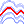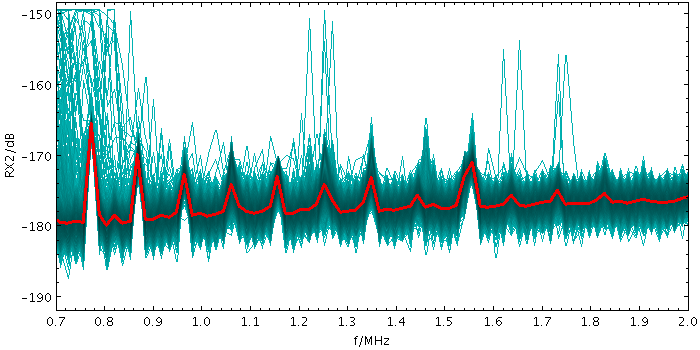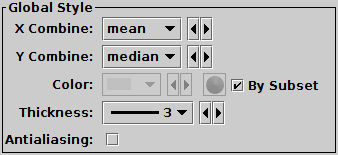Next Previous Up Contents
Next: StatMark Form
Up: Plot Forms
Previous: XYErrors Form

#### A.4.5.38 StatLine Form

The StatLine form (), available from the XYArray Layer Control, plots a single line based on a combination (typically the mean) of input array-valued coordinates. The input X and Y coordinates must be fixed-length arrays of length N; a line with N points is plotted, each point representing the mean (or median, minimum, maximum, ...) of all the input array elements at the corresponding position.

Note that because the X and Y arrays must be of a fixed size for all rows, and because combination is performed in both X and Y directions, this is typically only suitable for plotting combined spectra if they all share a common horizontal axis, e.g. are all sampled into the same wavelength bins. To visually combine spectra with non-uniform sampling, the ArrayQuantile form may be more useful.Example StatLine plotStatLine form configuration panel

The configuration options are:

X Combine
Y Combine
Defines how corresponding array elements on the X/Y axis are combined together to produce the plotted value. The following options are currently available:
• `mean`: the mean of the combined values
• `median`: the median of the combined values (may be slow)
• `min`: the minimum of all the combined values
• `max`: the maximum of all the combined values
• `q.01`: the 1st percentile of the combined values (may be slow)
• `q1`: the first quartile of the combined values (may be slow)
• `q3`: the third quartile of the combined values (may be slow)
• `q.99`: the 99th percentile of the combined values (may be slow)
• `stdev`: the sample standard deviation of the combined values
• `sum`: the sum of all the combined values
• `count`: the number of non-blank values
Color
Color of the plotted line.
Thickness
Thickness in pixels of the plotted line.
Antialiasing
Controls whether grid lines will be drawn antialiased (smoothed) or not. This option does not affect exported plots.

Next Previous Up Contents
Next: StatMark Form
Up: Plot Forms
Previous: XYErrors Form

TOPCAT - Tool for OPerations on Catalogues And Tables